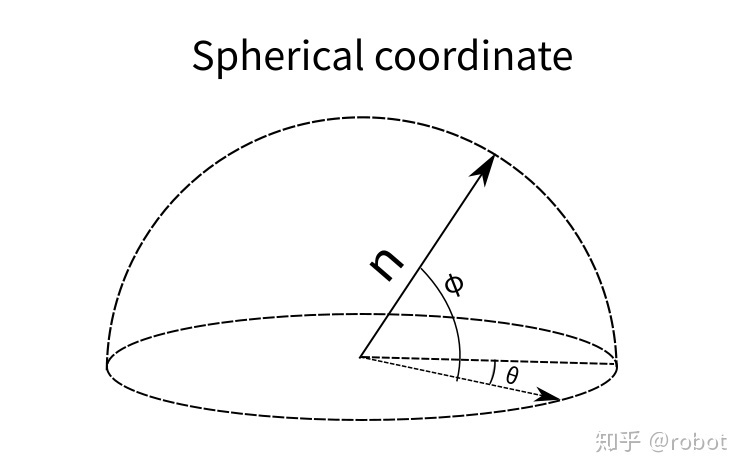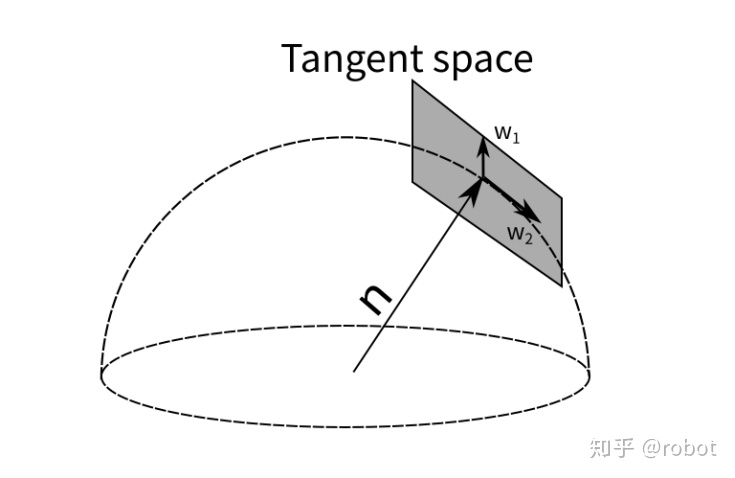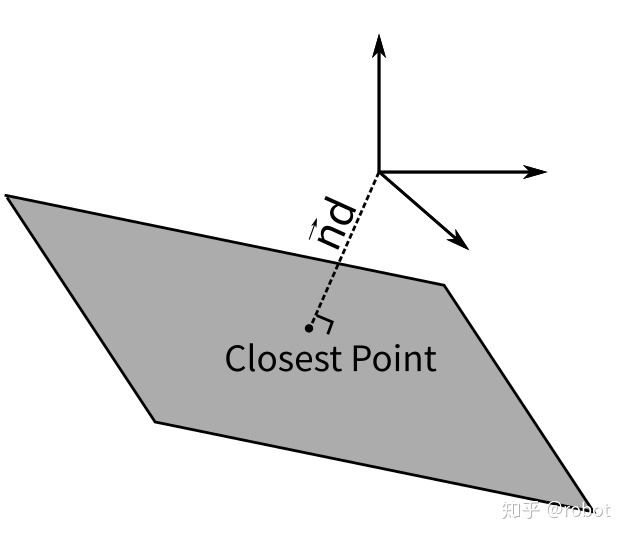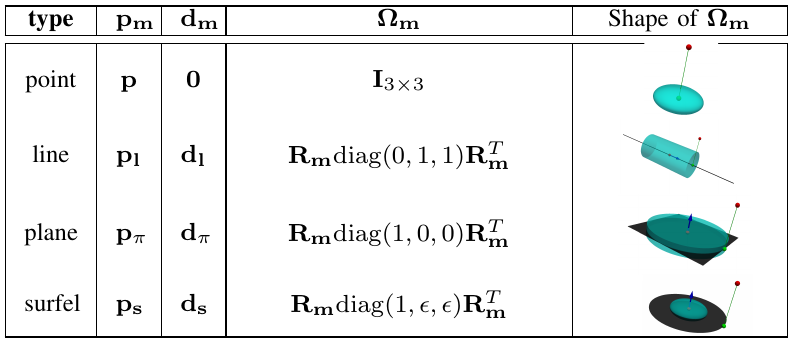[TOC]

• 一般表示形式

• 优化形式（避免过参数化）

# 3D Point

$P: \left[ X \; Y \; Z \right]^T$

• 3 DoF

ref:

• Global XYZ
• Anchored XYZ

## Inverse Depth

• Global inverse depth (spherical coordinates)
• has a singularity when the z-distance goes to zero
• 球坐标逆深度仅在xyz都趋近于0时才存在数值奇异，所以能用在全局坐标系
• Anchored inverse depth (MSCKF)
• 逆深度 + normalized UV (Bearing Vector)
• Anchored inverse depth (MSCKF single depth)
• 逆深度
• the the single depth from VINS-Mono

# 3D Line

$L: \left[ P_0 \; P_1 \right]$

• 4 DoF

ref:

• 过参数化 问题

# 3D Plane

$Ax + By + Cz + D = 0$

• 3 DoF

ref:

## Hesse

$\pi: \left(\mathbf{n}^{\top}, d\right)^{\top} \in \mathbb{R}^4$

## 球坐标$\pi: \left[ \theta \; \phi \; d \right] \in \mathbb{R}^3$

$\tau=(\theta, \phi, d)^{\top}= q(\pi)=\left(\theta=\arctan \frac{n_{y}}{n_{x}}, \; \phi=\arcsin n_{z}, \; d\right)^{\top}$

## 切平面GTSAM里面表示面的方式用切平面的方式来更新单位法向量，同样也可以被用来优化单位法向量。

## 最近点# Unified Representation# Reference

1. Unified Representation of Heterogeneous Sets of Geometric Primitives ↩︎

SLAM中的3D特征参数化表示
https://cgabc.xyz/posts/4294f23/

Gavin Gao

2022年3月6日